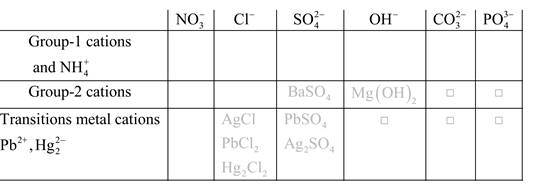Chapter 4, Problem 4QAPChemistry: Principles and Reactions

8th Edition
William L. Masterton + 1 other
ISBN: 9781305079373

Solutions

Chapter
SectionChemistry: Principles and Reactions

8th Edition
William L. Masterton + 1 other
ISBN: 9781305079373
Textbook Problem

Name the reagent, if any, that you would add to a solution of iron(lll) chloride to precipitate(a) iron(lll) hydroxide.(b) iron(lll) carbonate.(c) iron(lll) phosphate.

Interpretation Introduction

(a)

Interpretation:

The name of the reagent should be determined which is used to precipitate iron(III) hydroxide from the solution of iron(III) chloride.

Concept introduction:

Precipitation reactions: It is a type of chemical reactions where two soluble salts react with each other and formed different products, out of which one product must be insoluble in solution which is known as precipitate.Solubility of any compound is predicted by above solubility chart.

Blank boxes indicate no precipitate formation occurs which means soluble in dilute solution.

Boxes with grey small box will form precipitate from dilute solutions and boxes where formula is written this is a cation-anion combination that will form precipitate.

Explanation

The molecular formula of iron(III) hydroxide is Fe(OH)3

The molecular formula of iron(III) chloride is FeCl3

Here, group I salts can be used to form a precipitate with another metal.

Therefore, sodium hydroxide can be used to form precipitate Fe(OH)3.

The chemical equation is written as:

FeCl3(aq)+NaOH(aq)

Interpretation Introduction

(b)

Interpretation:

The name of the reagent should be determined which is used to precipitate iron(III) carbonate from the solution of iron(III) chloride.

Concept introduction:

Precipitation reactions: It is a type of chemical reactions where two soluble salts react with each other and formed different products, out of which one product must be insoluble in solution which is known as precipitate.Solubility of any compound is predicted by above solubility chart.

Blank boxes indicate no precipitate formation occurs which means soluble in dilute solution.

Boxes with grey small box will form precipitate from dilute solutions and boxes where formula is written this is a cation-anion combination that will form precipitate.

Interpretation Introduction

(c)

Interpretation:

The name of the reagent should be determined which is used to precipitate iron(III) phosphate from the solution of iron(III) chloride.

Concept introduction:

Precipitation reactions: It is a type of chemical reactions where two soluble salts react with each other and formed different products, out of which one product must be insoluble in solution which is known as precipitate.Solubility of any compound is predicted by above solubility chart.

Blank boxes indicate no precipitate formation occurs which means soluble in dilute solution.

Boxes with grey small box will form precipitate from dilute solutions and boxes where formula is written this is a cation-anion combination that will form precipitate.

Still sussing out bartleby?

Check out a sample textbook solution.

See a sample solution

The Solution to Your Study Problems

Bartleby provides explanations to thousands of textbook problems written by our experts, many with advanced degrees!

Get Started